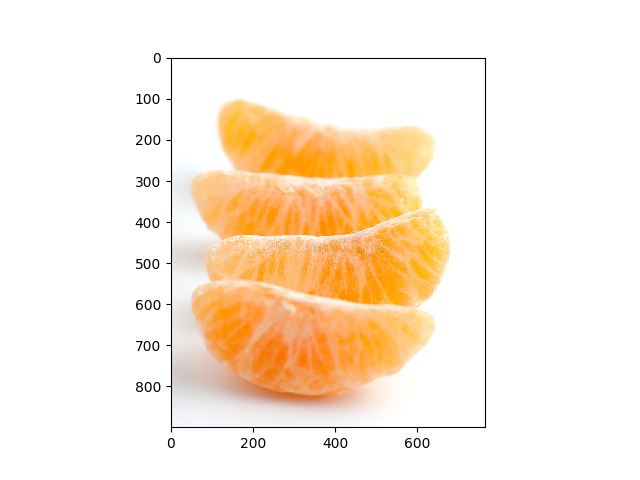# Classifying Segments Directly with a Neural Network¶

This is a basic example using a convolutional recurrent neural network to learn segments directly from time series dataOut:

```/home/circleci/miniconda/envs/testenv/lib/python3.8/site-packages/sklearn/utils/validation.py:67: FutureWarning: Pass memory=None as keyword args. From version 0.25 passing these as positional arguments will result in an error
warnings.warn("Pass {} as keyword args. From version 0.25 "
/home/circleci/miniconda/envs/testenv/lib/python3.8/site-packages/seglearn/transform.py:237: VisibleDeprecationWarning: Creating an ndarray from ragged nested sequences (which is a list-or-tuple of lists-or-tuples-or ndarrays with different lengths or shapes) is deprecated. If you meant to do this, you must specify 'dtype=object' when creating the ndarray
Xt = np.array([sliding_tensor(Xt[i], self.width, self._step, self.order)
N series in train:  105
N series in test:  35
N segments in train:  1783
N segments in test:  586
Accuracy score:  0.223549485206604

<matplotlib.image.AxesImage object at 0x7fe908543af0>
```

```# Author: David Burns

import matplotlib.image as mpimg
import matplotlib.pyplot as plt
from tensorflow.python.keras.layers import Dense, LSTM, Conv1D
from tensorflow.python.keras.models import Sequential
from tensorflow.python.keras.wrappers.scikit_learn import KerasClassifier
from sklearn.model_selection import train_test_split

from seglearn.pipe import Pype
from seglearn.transform import Segment

def crnn_model(width=100, n_vars=6, n_classes=7, conv_kernel_size=5,
conv_filters=3, lstm_units=3):
input_shape = (width, n_vars)
model = Sequential()

metrics=['accuracy'])

return model

X = data['X']
y = data['y']

# create a segment learning pipeline
pipe = Pype([('seg', Segment(width=100, step=100, order='C')),
('crnn', KerasClassifier(build_fn=crnn_model, epochs=1, batch_size=256, verbose=0))])

# split the data
X_train, X_test, y_train, y_test = train_test_split(X, y, test_size=0.25, random_state=42)

pipe.fit(X_train, y_train)
score = pipe.score(X_test, y_test)

print("N series in train: ", len(X_train))
print("N series in test: ", len(X_test))
print("N segments in train: ", pipe.N_train)
print("N segments in test: ", pipe.N_test)
print("Accuracy score: ", score)